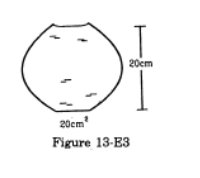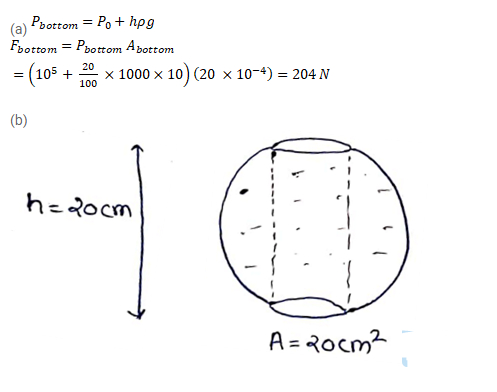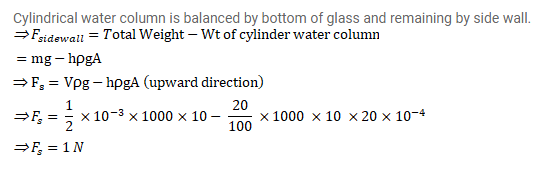# ﻿A glass of water has a bottom of area

Question:

A glass of water has a bottom of area $20 \mathrm{~cm}^{2}$, top of area $20 \mathrm{~cm}^{2}$, height $20 \mathrm{~cm}$ and volume half a litre.

(a) Find the force exerted by the water on the bottom.

(b) Considering the equilibrium of the water, find the resultant force exerted by the sides of the glass on the water.

Atmospheric pressure $=1.01=1000 \mathrm{~kg} / \mathrm{m}^{3}$ and $\mathrm{g}=10 \mathrm{~m} / \mathrm{s}^{2}$. Take all numbers to be exact.Solution: